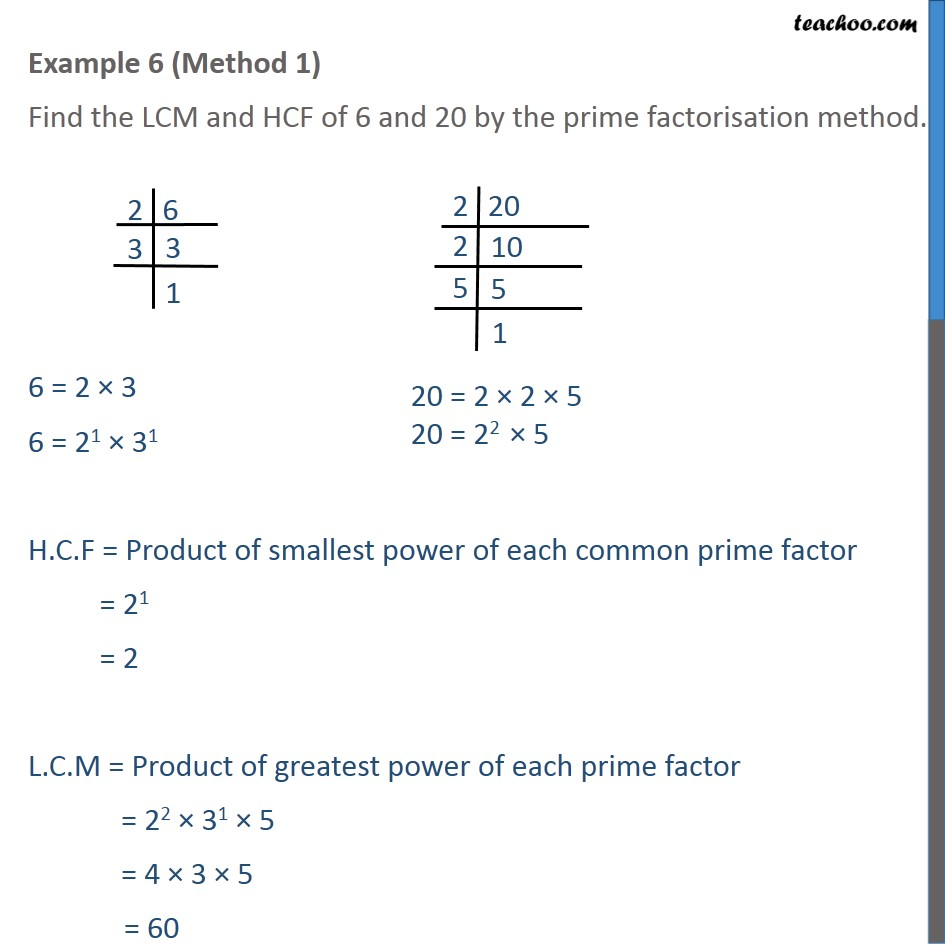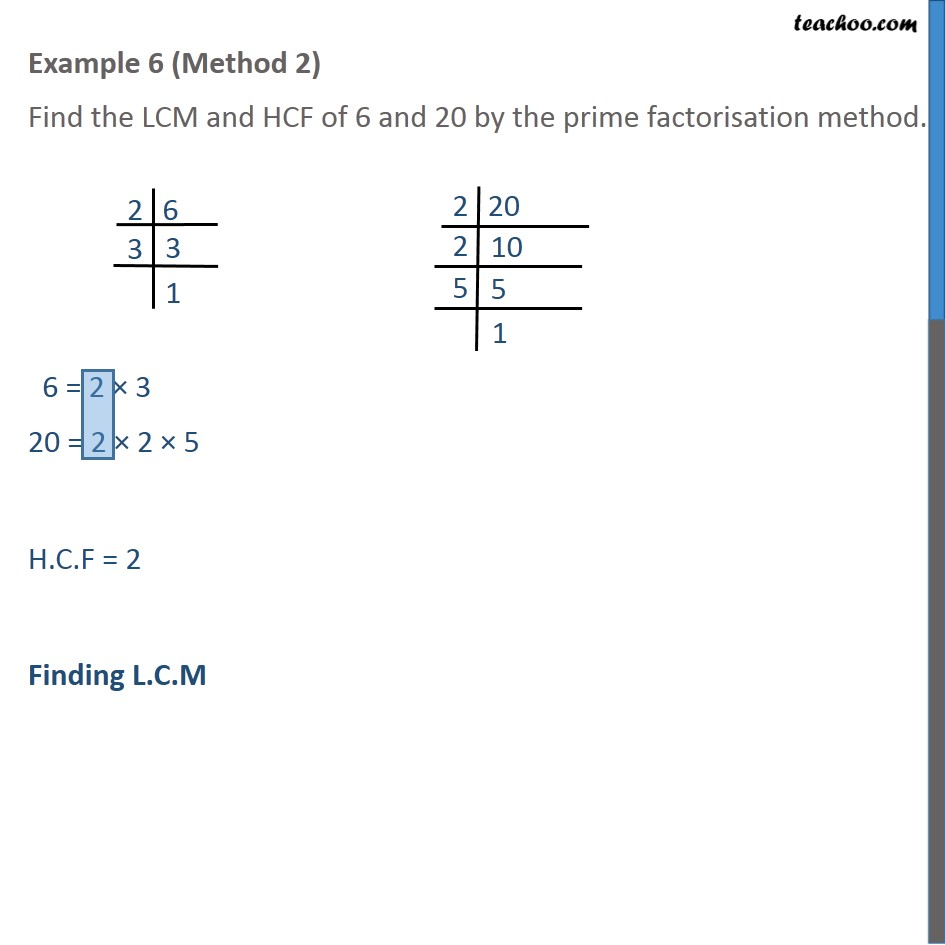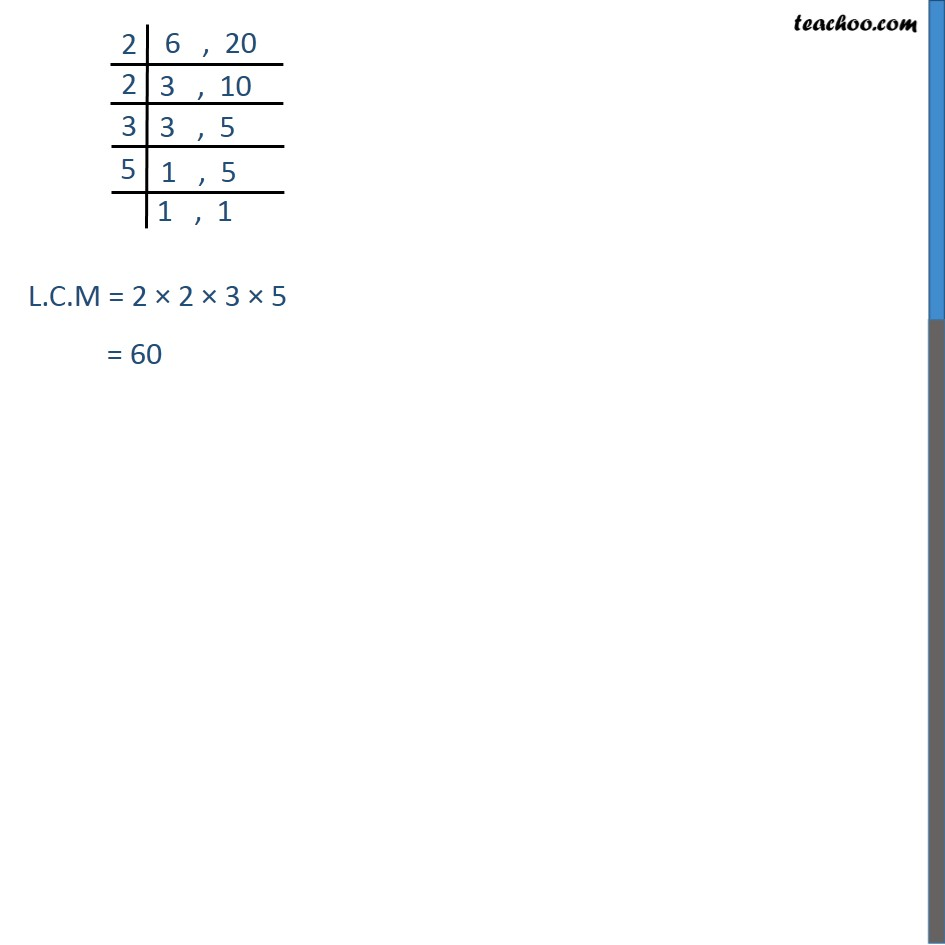Check Important Questions and Chapter Summary -https://you.tube/Real-Numbers-Class-101. Chapter 1 Class 10 Real Numbers
2. Concept wise
3. LCM/HCF

Transcript

Example 6 (Method 1) Find the LCM and HCF of 6 and 20 by the prime factorisation method. 6 = 2 3 6 = 21 31 H.C.F = Product of smallest power of each common prime factor = 21 = 2 L.C.M = Product of greatest power of each prime factor = 22 31 5 = 4 3 5 = 60 Example 6 (Method 2) Find the LCM and HCF of 6 and 20 by the prime factorisation method. 6 = 2 3 20 = 2 2 5 H.C.F = 2 Finding L.C.M L.C.M = 2 2 3 5 = 60

LCM/HCF